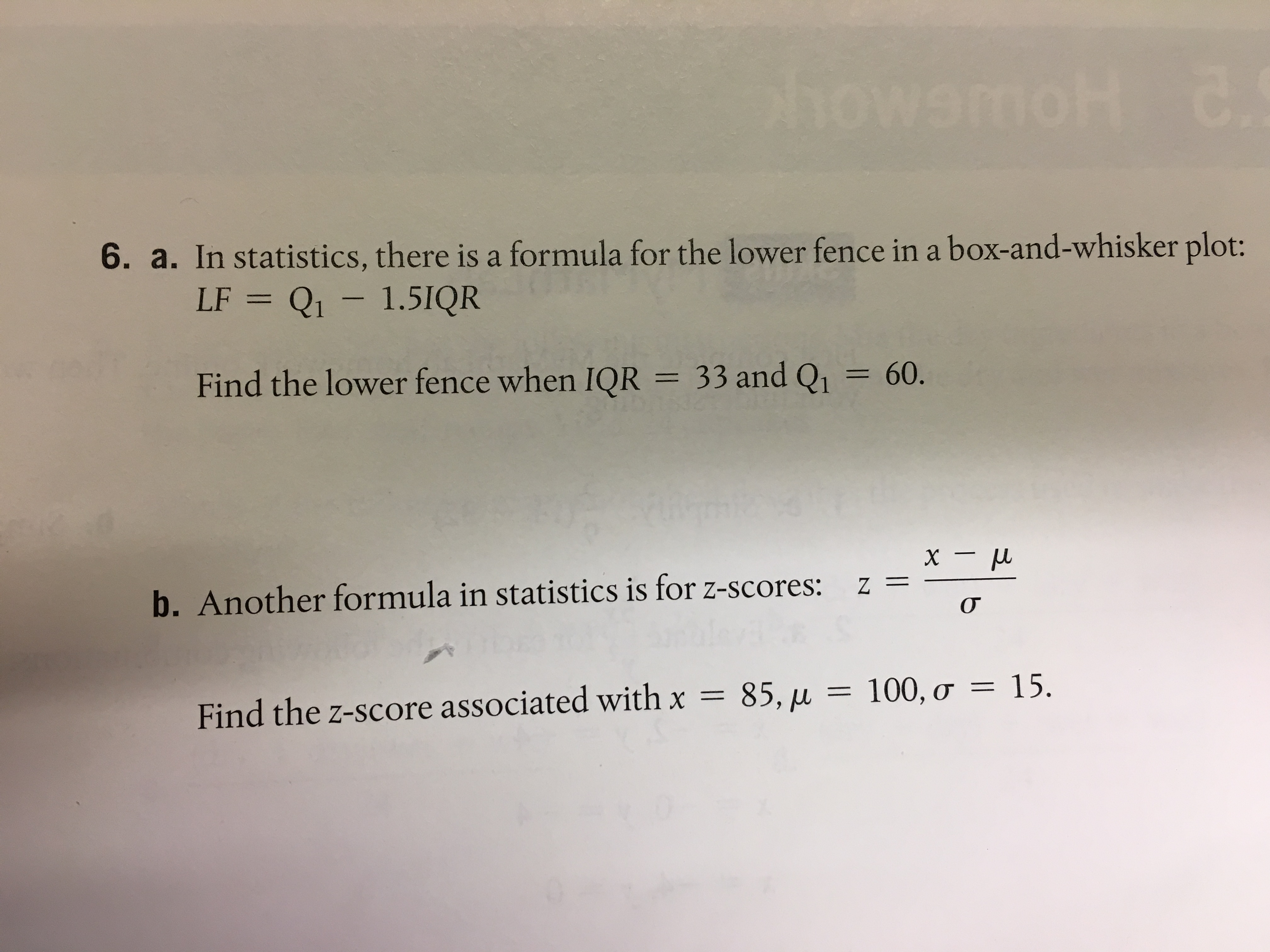6. a. In statistics, there is a formula for the lower fence in a box-and. whisker plotFind the lower fence when IQR 33 and Q160.xμb. Another formula in statistics is for z-scores:z=-Find the z-score associated with x85, μ100, σ15.

Questionhelp_outlineImage Transcriptionclose6. a. In statistics, there is a formula for the lower fence in a b ox-and. whisker plot Find the lower fence when IQR 33 and Q1 60. xμ b. Another formula in statistics is for z-scores: z= - Find the z-score associated with x 85, μ 100, σ 15. fullscreen
Step 1

Formula used:

Step 2

a)Given thatIQR = 33 and  Q1 =60

So value of lower fence is

Step 3

b) Given that

&n...

Want to see the full answer?

See Solution

Want to see this answer and more?

Our solutions are written by experts, many with advanced degrees, and available 24/7

See Solution
Tagged in

Algebra